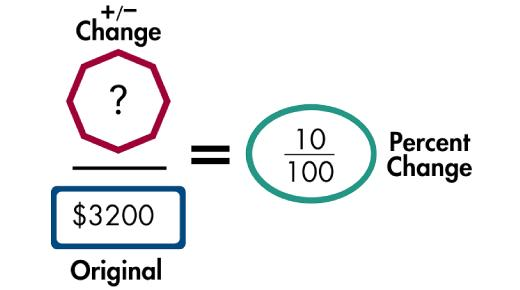# Percent Equation: Can You Pass This Test? Math Trivia Quiz

9 QuestionsSettings.

Related Topics
• 1.
Using the Percent Equation: Solve and Show your Work: 3 is 12 % of what number?
• 2.
Using the Percent Equation: Solve and Show your Work: 24 is what percent of 120?
• 3.
Using the Percent Estimation: Solve and Show your Work: 21% of 90
• 4.
What number is 40% of 82?
• A.

.40 multiplied by 82

• B.
• C.
• D.
• 5.
Check the following that could be applied to percent estimation for the following problem: 27% of 125
• A.
• B.
• C.
• D.
• 6.
A cell phone survey found  75% of the age 18-25 gave up their home phone and only use a cell phone.  If 4, 264 people only use a cell phone,  about how many people were surveyed?   Determine the best option(s) you would use to determine the answer.
• A.

Percent Estimation

• B.

Percent of a number

• C.

Percent Equation

• D.

Percent Proportions

• 7.
Using percent Proportion, check all the ways this problem can best be represented. What number is 25% of 180?
• A.
• B.
• C.
• D.
• 8.
40% of what number is 82?
• A.

206

• B.

210

• C.

310

• D.

205

• 9.
Determine what is the correct demonstration of percent equation for the following situation:  Molly had how many Legos in her box if it was 88% of 300.
• A.
• B.
• C.
• D.